# NP

(diff) ← Older revision | Latest revision (diff) | Newer revision → (diff)

One major goal of complexity theory is to obtain precise bounds on the number of operations performed by algorithms for solving a particular problem. This purpose splits into two directions: the design of specific algorithms leads to upper bounds on the computational complexity of a problem; the analysis of all permitted algorithms together with the structure of a problem can provide lower bounds (cf. also Algorithm; Algorithm, computational complexity of an).

If both bounds match, the ideal situation of an optimal algorithm is reached. However, in many situations such optimal bounds are not (yet) available. Consequently, one is tempted to give at least a classification of problems in terms of statements like: one problem is at least as hard to solve as another one. This leads to the notions of reducibility and completeness.

Consider a computational model for uniform algorithms over a set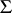. Two prominent examples are

1) The Turing machine: here,is a finite alphabet, typically. Operations used are bit operations on strings over(see [a2]).

2) The real Turing or Blum–Shub–Smale machine (BSS model): here,is the set of real numbers. Operations used are the arithmetic operations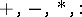, together with a test-instruction "x≥ 0?" for an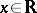(see [a1]).

A decision problem is a subsetof the set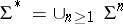. An algorithm solvesif it computes the characteristic function ofin. In order to speak about complexity, a size measure for a problem instance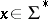as well as a cost measure for algorithms must be introduced. In the above settings, the size ofis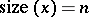, whereas the cost or running time of a corresponding algorithm is the number of executed operations. (In particular, for computations over integers the Turing model uses a logarithmic size measure together with bit-costs whereas in the BSS model one exploits algebraic measures.)

Next, complexity classes (of decision problems) can be defined by bounding the running time of an algorithm in the size of a problem instance. Of particular importance is the classof problems solvable in polynomial time. A problem is inif it can be decided by an algorithm the running time of which is bounded by a polynomial function in the input size.

For example, the linear programming problem is inover the integers in the Turing model (see [a4]). It is not yet (1998) known to belong toover the real numbers.

Problems in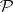are considered to be efficiently solvable. However, for a large class of problems it can only be shown that there exist fast verification procedures instead of fast decision procedures. The latter is formalized by defining the class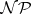.

A decision problemis in the classif there exists an algorithmsuch that the following holds: As inputtakes an element(the current problem-instance) together with an additional "guess"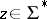(representing a possible proof of "x S" ). If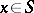, there must exist a suitable guess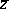such thaton inputaccepts in polynomial time with respect to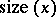. If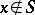, noexists for which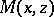accepts.

Such algorithms are called non-deterministic since there is no information available on how to obtain a correctefficiently.

Note that; the question whether equality holds is the major open (1998) problem in complexity theory.

## Examples.

### Turing model.

Given a graphovernodes, theproblem asks for the existence of a Hamiltonian circuit (cf. also Graph circuit). A fast verification procedure is given by guessing a permutation of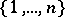and checking whether it represents such a circuit of. Thus,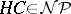over.

### BSS-model.

Given a polynomial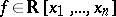of degree, the problem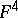asks for the existence of a real zero for. A fast verification procedure is given by guessing a possible zero, plugging it intoand checking whether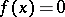. Thusover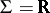.

### Remarks.

For the above examples (and for many more), no optimal complexity bounds are known. Nevertheless, among all other problems within the correspondingclass they have a particular status. A decision problemis called reducible in polynomial time to another problemif there exists a polynomial-time algorithmmappingto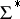such that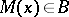if and only if. Problemis called-hard if all problems inare reducible toin polynomial time. It is called-complete if, in addition,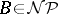holds.

## Examples.

### Turing-model.is-complete over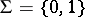(cf. [a2]).

### BSS-model.is-complete over(cf. [a1]).

### Remarks.

If any-hard or-complete problem would be solvable in polynomial time, then. This is the substantiation why-complete problems are of particular interest in complexity theory.

Knowing the completeness or hardness of a problem does not remove the necessity of solving it. There is a huge literature on that issue in connection with many complete problems. As starting point with respect to the above-mentioned problems in graph theory and polynomial system solving, see [a3], respectively [a1].

Finally, it should be noted that hardness and completeness notions can also be defined with respect to other resources, such as space or parallel computational devices (cf. [a2]).

How to Cite This Entry:
NP. Encyclopedia of Mathematics. URL: http://encyclopediaofmath.org/index.php?title=NP&oldid=15503
This article was adapted from an original article by Hubertus Th. JongenKlaus Meer (originator), which appeared in Encyclopedia of Mathematics - ISBN 1402006098. See original article